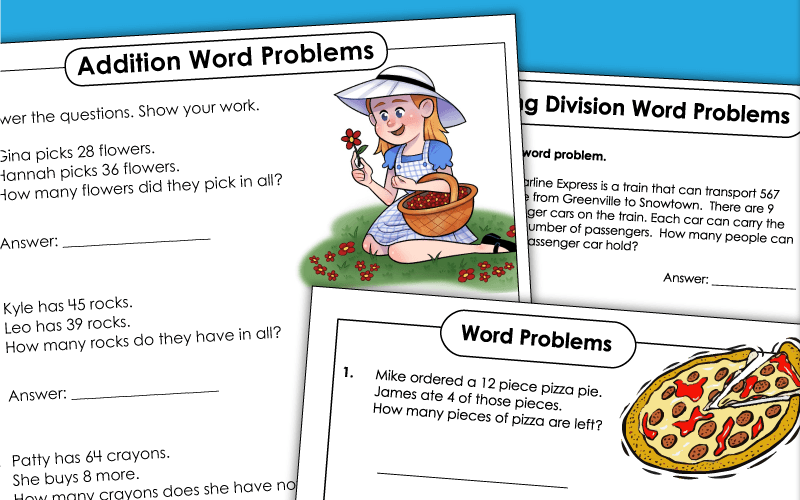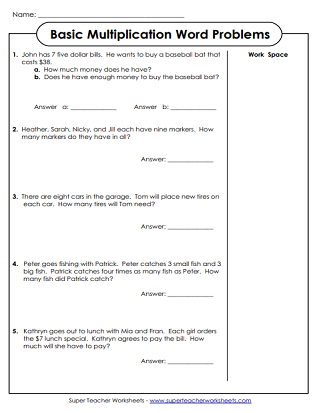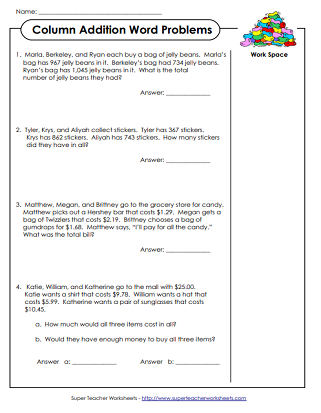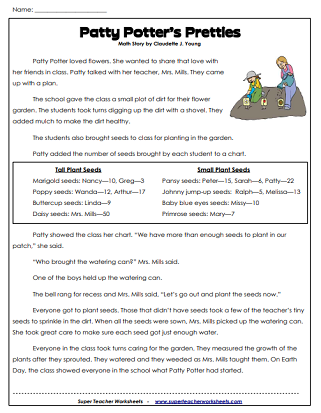# Math Word Problems

Word problems (or story problems) allow kids to apply what they've learned in math class to real-world situations.  Word problems build higher-order thinking, critical problem-solving, and reasoning skills.These two-digit word problems do not require students to regroup (carry) numbers across place values.
Solve these two-digit addition word problems. All problems require carrying (regrouping).
Read and solve these 3-digit addition word problems. There is plenty of space for students to show their work.
These word problems require students to find the sums of four-digit addends.

## Subtraction

Subtraction story problems that require knowledge of borrowing. (Includes 2 and 3-digit numbers)
Subtract the amount each person gives the cashier from the amount due. Requires subtraction across zero.
Subtract the cost of each item from the amount paid to the cashier. (example: \$5.00 - \$1.34)
These multiple-step problems require students to first add the cost of products, then subtract to find the change.

This printable worksheet focuses on basic addition and subtraction. The numbers in each problem are single-digit.
This worksheet has four word problems on it with both addition and subtraction problems on it with facts up to 20.

## Multiplication

Word problems help students to review 0 - 9 basic facts.
Several two-digit by one-digit multiplication word problems. (example: 22 x 8)
A sheet of three-digit by one-digit multiplication word problems. (example: 340 x 7)

## Division

These word problems require students to use long division with 3-digit dividends.

## Other

These word problems require students to compare two fractions and determine which is greater.
Read the word problems and determine how much money each person has.
Find the average of the given numbers in each word problem. Students will need to find the sum of the numbers, then divide by the number of addends.
Multiple-Step Word Problems

Word problems where students use reasoning and critical thinking skill to solve each problem.

Math Word Problems (Mixed)

Mixed word problems (stories) for skills working on subtraction,addition, fractions and more.

Math Worksheets - Full Index

A full index of all math worksheets on this site.

## Images of WorksheetsMy Account
Site Information